## General Chemistry

Learn the toughest concepts covered in Chemistry with step-by-step video tutorials and practice problems by world-class tutors

16. Chemical Equilibrium

# Chemical Equilibrium

Most chemical reactions never go to completion where all the reactants are converted into products. Instead these reactions reach a chemical equilibrium

Chemistry & Equilibrium
1
concept

## Understanding Chemical Equilibrium3m
Play a video:

A reaction reaches equilibrium once the rate of the forward reaction equals the rate of the reverse reaction. Once at equilibrium there is no net change in the concentration of reactants or products.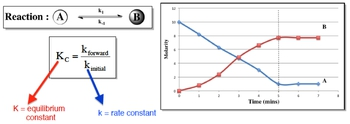Whereas the rate constant, k, is affected by factors dealing with the rate of reaction, the equilibrium constant, K, is affected by only temperature.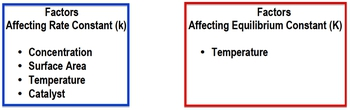2
Problem

Which one of the following statements does not describe the equilibrium state?

a. While at equilibrium, a dynamic process is still occurring.

b. The concentration of the reactants is equal to the concentration of the products.

c. The concentration of the reactants and products reach a constant level.

d. At equilibrium, the net concentration of all species is not changing.

e. All are true.

The Equilibrium Constant K

Associated with any reaction at equilibrium is the equilibrium constant K. Its numerical value determines if reactants or products are more greatly favored within a reaction.

3
concept

## The Equilibrium Constant Concept 12m
Play a video:
Hey, guys, In this brand new video, we're gonna take a look at the equilibrium. Constant. Now we're going to say that the variable we usedto illustrate our equilibrium. Constant is the variable K. We're gonna say K is a number equal to the ratio of products to react. It's at a given temperature. Why do I say at a given temperature? Because we're gonna say that temperature directly effects are equilibrium, constant K increasing the temperature will increase our K value. Decreasing our temperature will decrease r k value. Now we're gonna say it's a ratio of products to react in. So we're gonna say here that K equals products, overreact INTs. Now we're gonna say that K is important because it it's magnitude tells us how far to the left or to the right are. Chemical reaction is at a given temperature. So we're gonna say if K is greater than one than products, our favorite overreact. It's and think about it. This makes sense because we said K equals products. Overreactions. So let's say our products are 10 on our reactant are one k equals 10 definitely greater than one. We're gonna say when products are favorite over our reactant. That means we're making more products. How do we make more products by going in the forward direction so the four direction would be favored now in the opposite way if K is less than one? So let's say we have. In this case, products are one, but our reactions air 10 we have a K less than one. So in this case, if K is less than one than react INTs our favorite over products, which means our reaction is heading in the reverse direction so the reverse direction would be favored. So I just remember these two differences when K is greater than one when K is less than one Now, what if we say Kay was equal toe one? We know that this is products, overreact INTs. So that means that our products and reactant would have to be equal. Let's say they're both 10. 10/10 is one. So when K equals one, we're gonna say both are reacting in our product. Amounts are equal to one another. Now we're gonna say the equilibrium constant K takes into account all the states of matter except to it doesn't look at solids, and it doesn't look at liquids. It ignores those two states for matter

The equilibrium constant K is a ratio of products to reactants. It only deals with gaseous or aqueous compounds.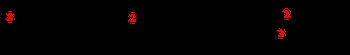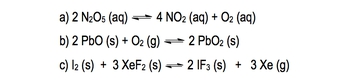4
example

## The Equilibrium Constant Example 13m
Play a video:
so it looks at it only cares about really gasses and also acquis a quiz Just mean that the solvent is water. So a compound is dissolved in water. It would be a quiz. Now, knowing this, let's take a look at at the following example it says right, the equilibrium expression for the following reaction. Here we have to end 205 Acquis gives us four and 02 acquis plus O two. Acquis. It's important to look at the phases because remember, if it's a solid or liquid, we ignore it. Now we're gonna say here K equals products, overreact INTs So we're gonna have our products is n 02 on. Remember, you have to use the coefficients in these calculations. The number in front of No. Two is a four, so that four will become the power. So it's gonna be no to to the fore times 0202 just has a coefficient of one in front of it, which we don't have to show divided by and 205 again, the coefficient in front of end 12 5 is too. So that becomes a power. So we would say that this represents our equilibrium, expression or are equilibrium equation Same thing. Equilibrium, expression, equilibrium, equation. Now that we've seen this one, let's look at B okay, for be we're gonna say here, we're gonna ignore this compound because it's a solid and we're gonna ignore this compound because it's a solid here. We're gonna say K equals products. Overreacting. It's like before, but here's the thing we're gonna say we don't have any products available, but you have to put something on top. We're gonna say it's equal toe one solids and liquids are ignored, and in place of them, we're gonna put one. We're going to say this because things such as pressure don't really affect solids and liquids like they do gasses and acquis compounds. That's why they're equal toe one because their numbers being held constant. Okay, so we're gonna replace solids and liquids with the number one on on the bottom. We'd have 02 now differently your professor could give you this is we know there's a one here, so this could also be or in verse one. So be aware of both types of answers. Both are correct. Both are saying the same thing in verse. One just means one over whatever it ISS for the next one. Again, we ignore solids. The only thing that we don't ignore is this gas. So here K equals products. Overreact INTs, so just equal x e cute because of the three over one. But we don't need to put the one because anything over one is the same exact thing. So that would be our equilibrium expression. So it was pretty simple. But remember the differences in B. N. C. We ignore the solids and liquids. Now that you've seen this, I want you guys to attend to answer a question that follows this one. The practice one. Here. I want you to tell me who's greater in amount is a products or is a reactant from that. You have to remember, what do we say about K when it's greater than one who's favored when it's less than one who's favored when it's equal toe? One who's favored remembering that will be a great way for you to approach this problem. Good luck, guys.
5
Problem

State which is greater in amount:reactants or products, based on the given equilibrium constant, K.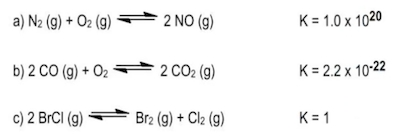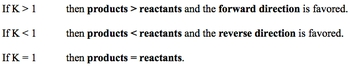6
Problem

The decomposition of nitrogen monoxide can be achieved under high temperatures to create the products of nitrogen and oxygen gas.

6 NO(aq) ⇌ 3 N2(aq) + 3 O2(aq)

a) What is the equilibrium equation for the reaction above?

b) What is the equilibrium expression for the reverse reaction.

7
Problem

The equilibrium constant, K, for 2 NO (g) + O2 (g) ⇌ 2 NO2 (g) is 6.9 x 102

What is the [NO] in an equilibrium mixture of gaseous NO, O2, and NO2 at 500 K that contains 1.5 x 10 –2 M O2 and 4.3 x 10 –3 M NO2?

Kp and Kc

When dealing with equilibrium units in terms of pressure the equilibrium constant of Kp must be used, but if the equilibrium units are in molarity then we must use the equilibrium constant of Kc

8
concept

## The Different Types of Equilibrium Constants3m
Play a video:
Hey, guys, In this new video, we're gonna take a look at the different types of equilibrium. Constants. Now, one thing we're gonna realize by going through chemistry one in chemistry to is that K is a very, very popular variable. We have it everywhere. We have our rate constant K. We have actually a K for acids and bases. We call them K a N K B K A. Is called our acid association constant, whereas K B is called Are based association Constant. Now we're talking about different types of equilibrium. Constants. So K is really, really popular. And when it comes to calculations in chemistry now we've talked about the equilibrium constant. Up to this point, we've said that it's equal toe products. Overreact. It's and we should realize, is that we have different types of equilibrium constants based on the units that we're using. Now we're going to say when dealing with gas is, we usually use the equilibrium constant K p. We're gonna say KP uses the partial pressure unit off atmospheres, and we're gonna say when dealing with a quiz salutes remember, acquis means that water is dissolving my compound. So when we're using Equus salutes, we tend to use the equilibrium constant Casey. Now Casey uses the concentration unit off polarity E. Remember, Polarity is moles over leaders. And also remember, polarity is the same thing as concentration and the same thing as Saul. You bility. So we've seen that we have K, p and K C now, and we're going to say that these two are related together. By this equation, KP equals Casey rt to the Delta end here. We're going to say that our is called our gas constant and it equals 0.8 to 06 leaders times atmospheres over moles Times K. Now remember, there's another are variable out there. It's 8.314 Jules over moles times K. But we only use that one when we're dealing with energy, velocity or speed. In this case, we're not dealing with energy, velocity or speed. We're dealing with equilibrium concentrations. So that's why we're using this particular are we're gonna say that t represents our temperature in Kelvin and then we're gonna say, most importantly, is our delta and exponents. Delta End equals moles off gas as products minus moles off gas again. Remember gas as react INTs. So we started out with the broad idea of an equilibrium constant equilibrium, constant K. But now we've delved in deeper to K, P and K C.

Kp and Kc are related to one another by the following equation below: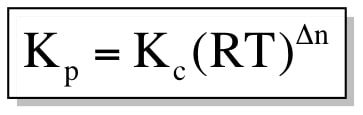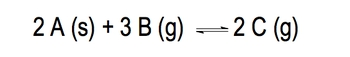9
example

## For the reaction above, the Kc = 4.9 x 10-9 at 25oC3m
Play a video:
now knowing the connection between them. Let's take a look at the following example and try to answer the question. So in this question, it says for the following reaction to a solid plus three. B gas gives us to see gas. This equation has a Casey value off 4.9 times 10 to the negative nine at 25 degrees Celsius. Now we're saying which of the following statements is true, so I'm gonna take a look at the first one. It says the reaction is favored in the in the Ford direction. Remember, we've seen this type of terminology before. When is it favorite In the four directions? It's on Lee favorite in the four direction when K is greater than one. But we can see that based on the Casey, Value K is a lot smaller than one, so the four direction would not be favored. Next, we're gonna say the concentration of the products is greater than the concentration of the reactant. Again, this would Onley happen when K is greater than one. So this is also out. See, the reaction is favored in the reverse direction. This is true when K is less than one. And here are K. C is times 10 to the negative nine. So it's incredibly small, So the reverse direction is highly favored, so C is definitely true. Now let's take a look at the last one D. It says the value of KP will be larger than the value of K C. Now we know that the equation that connects them is k p equals K. C are tee to the Delta end. We know that, Casey, I told you, is 4.9 times 10 to the negative nine. Our is our gas constant, which doesn't change. Our temperature is 25 degrees Celsius. So we add 2 73 15 to that to get to 98. 15 Calvin when it's times to 98.15. Calvin, remember, we have to figure out what Delta and is here. So remember Delta and is gonna look at our e r products as gas. We have two moles of gasses products minus this Here is a solid. We don't look at solids. We're only looking at the gas minus three moles of gas so delta and is minus one. So when we punch that into our calculators we're gonna get back to 0.0 times 10 to the negative 10 and you can see that KP is actually smaller than Casey, So D is also false. Hopefully, guys were able to remember the relationship for our equilibrium. Constant its products overreact. INTs. When K is greater than one, the four direction is favored, and our products are greater in amount than react. It's when K is less than one than the reverse is favored, and our reactant will be greater than our products. Now that we've seen how to do this one, I want you guys to attempt to do this one on your own. So in this question, I want you to figure out what Casey is. I give you all the equilibrium amounts for each compound so we can use products overreacting to help us get to Casey. See if you guys can answer this, then come back and click on the explanation button and see what I do to solve the same exact question. Good luck, guys.
10
Problem

Methane (CH4) reacts with hydrogen sulfide to yield hydrogen gas and carbon disulfide, a solvent used in the manufacturing rayon and cellophane. What is the value of Kc at 1000 K if the partial pressures in an equilibrium mixture at 1000 K are 0.20 atm methane, 0.15 atm hydrogen sulfide, 0.30 atm carbon disulfide and 0.10 atm hydrogen gas?

CH4 (g) + 2 H2S (g) ⇌ 4 H2 (g) + CS2 (g)

Determination of the value for Δn can help us determine if Kp is greater than, less than or equal to Kc

11
Problem

In which of the given reactants is Kp greater than, less than and equal to Kc?

a) SO3 (g) + NO (g) ⇌ SO2 (g) + NO2 (g)

b) P4 (s) + 5 O4 (g) ⇌ P4O10 (s)

c) 4 NH3 (g) + 3 O2 (g) ⇌ 2 N2 (g) + 6 H2O (g)

12
Problem

Given the hypothetical reaction 2 A (s) + ? B (g) ⇌ 3 C (g), Kp = 0.0105 and Kc = 0.45 at 250 degrees Celsius. What is the value of the coefficient of B?

Hess’s Law and the Equilibrium Constant K

The old concept of Hess’s Law can be applied to our new concept of the Equilibrium Constant K.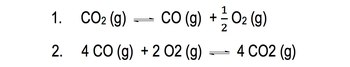13
example

## The equilibrium constant K for the first reaction is 6.83 x 10-12 at 1000 K3m
Play a video:
Hey, guys, In this new video, we're gonna take a look at Hess's law. At least kind off. Now, we're gonna say that when it comes to Hess's law, we learned that the total entropy change. So total delta h of a reaction by taking into account each individual step. Now, we're gonna do the same exact thing. Except instead of using Delta H, we're gonna use our equilibrium, constant K. So they pay very close attention to what I do here for these two particular examples. Now we're gonna say the equilibrium constant for the reaction. This reaction here is 6.83 times 10 to the negative, 12 at 1000 Kelvin. Now I'm asking you to find a new K for this new reaction. Now, what you should realize here is that both of these reactions are the same. They both have CO two. They both have CEO. They both have 02 for the same exact equation. The only difference here is I changed and manipulated the one on the top so that it now looks like this one. So what we have to figure out is what changes that I do and what effect will that have on the equilibrium? Constant K. So we're gonna say first Co two was a reactant, but now it's a product. These guys were products. Now they're reacting. So it looks like I reverse the reaction and just realize when I reverse a reaction, K becomes the reciprocal. So K was 6.83 times 10 to the negative 12 while now it becomes 1/6 0.83 times to the negative 12 it becomes the reciprocal of whatever it waas. Now, that's one change that I did. The other change that I did. It looks like I multiplied by four. Because here this CO two has a four. Now, this CEO has a four now. And when we do four times a half the two councils out with this four and that's how we got this to here. Now, when you multiply by a number, that number becomes the power. So this is gonna become to the fourth. So it's gonna become 1/6 0.83 times 10 to the negative, 12 to the fourth. Okay, so when you multiply by number, it becomes a power. So what we're gonna do here is just solve for what's inside 1st. 1/6 0.83 times, 10 to the negative 12. That gives us 1.46 times 10 to the negative 11. Well, actually to the positive 11. And now that's to the fourth. So that's going to give me 4.60 times to the 44 don't. So that will be our answer here. So remember, if you reverse the reaction, then we're gonna say that becomes the reciprocal one over whatever. When we multiply by a number, that's gonna become the power you multiply by five, it becomes power five. Let's say we divided by a number. Let's say we divided by three. Well, just remember, if you're dividing by three, that is the same as multiplying by one third. And if you're multiplying by one third, then one third becomes the power, let's say you have to divide by two. If you're dividing by two, that's the same thing as multiply by a half. And in that way half becomes the power. Okay, so that's how we get around the dividing aspect of this

Reversing a reaction gives us the reciprocal of K, while multiplying a reaction by a value makes it the power for K.

14
example

## Calculate the rate constant, Kc, for the reaction below6m
Play a video:
So here this one's a lot more complicated. What we're gonna say here is we need to find the K value Casey value for this equation here. We're gonna call this K C four. This will be K C 12 and three. Since we're gonna need some room to do this, guys, I'm gonna take myself out of the image so we have more room to work with. What? So our best strategies for a question like this is, since we're trying to isolate that equation above, we have to look and see. Where do we see each one of these elements? So what we need to do first is find h gas and we're gonna notice that we see h gas right here. Now we have to manipulate this one in red, so it matches up with this one in blue. So what to changes? Do I need to do to it first? The H in red is a product, but we needed to be reacted. So we need to reverse the reaction once we reverse it. We also need to realize that there's a two in front of this H But over here in the blue box there, Isn't it a two. So we need to divide by two. And we just said dividing by two is the same thing as multiplying by half. So we have to reverse it and multiply by a half. Remember, dividing by two is the same thing is multiplying by half. So now our equation becomes H gas gives me half H two gas. When we reverse it, Que becomes 1/11 0. and because we multiplied by a half, half becomes the power. And just remember, half power really means square root. Okay, we're going to say that this right here got manipulated so we no longer care about it. And remember, we did all of this so that the red H gas that we have matches up with the H gas in the blue box. We're basically trying to manipulate these three equations so that they look like the compounds in the blue boxes above next we're gonna see where do we see br br gas here in the blue box? It's a reactant, but over here it's a product also here in the blue box, it doesn't have a coefficient in front of it. But here it has a two So the two changes are the same We need to reverse it So it becomes a reacted to and we need to divide by two which is the same thing as multiplying by a half. So this becomes BR gas gives me half br to gas So we reversed it So it becomes the reciprocal and we multiplied by a half so half becomes the power Remember half powers, the same thing A square root Finally hbr where do we see HBR? Luckily, we c h b r and only one place We see it right here as a product So they're both products so that's good. So there's no need to reverse but there's a two in front of that HBR So what do we need to do? We need to divide by two or same thing is multiplied by a half So this is gonna become half h two gas plus half br to gas gives me hbr Since I multiplied by half this is to the half power half powers, the same thing a square root. Okay, What we're gonna do next is we're gonna cross out intermediates things that look alike but our but is a product in one equation and are reacting in another. So we're gonna say here this h two is a product. This H two is a reactant. So they're going to cancel out this half Br two is a product, but this half br two is a reactant. They cancel out whatever doesn't get canceled. Out comes down. So what did you canceled out? H gas didn't get canceled out be our gas didn't get canceled out hbr gas and get canceled out and look, the equation we just isolated matches up with the equation above. Now all we have to do is we're gonna say K c four for missing equation equals K C one times k c two times k c three. So, basically, all we have to do here is we have to multiply each one of these right here. So multiply this times this times this multiplying all three of them will give us a K C four value of 4526. Hopefully, you guys were ableto follow along as we did this problem. Just realize the equation with the missing Casey value is the one we have to look at And we just looked compound by compound, look to see Where do we find them? In the given equations below When when were able to isolate them, you would just multiply all their K values together. And that will give us the overall K value for this equation we're looking for in the first place. So I just remember the tips that we did. If you reverse it, K becomes a reciprocal. If you multiplied by a number that becomes the power. If you divide by number, then it becomes one over that number as a power. So if you divided by five would be multiplying by 1/5. If you divided by four, it be like multiplying by 1/4. Those would become the powers. Just remember those small little principles, and you'll be able to get these questions correct.
15
example

## Hess's Law & Equilibrium Constant K3m
Play a video: